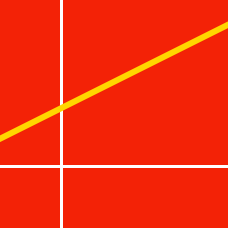Geometry

# Linear Equations - Parallel and Perpendicular Lines

What is the $y$-intercept of the line that is parallel to the line $5x - y - 3 = 0$ and passes through the point $(-9, 16)$?

Two lines $y=-\frac{1}{4}x+3 \text{ and } y=ax-9$ are perpendicular to each other. What is the value of $a ?$

The $2$ lines

$mx-4y-5m+3=0, \\ 12x+(m+17)y+7m-29=0$

are perpendicular to each other for some real number $m$. What is $m$?

If the two sets in the Cartesian plane  \begin{aligned} A &= \{(x,y) \mid x+ay+1=0\} \\ B &= \{(x,y) \mid 3x-by+1=0\} \end{aligned}  satisfy $A \cap B=\emptyset,$ what is the value of $\frac{b}{a}?$

Line $AB$ passes through two points $A=(7,\ 10)$ and $B=(10,\ 9)$. If a line is perpendicular to line $AB$ and passes through points $(-5,\ 12)$ and $(2,\ a)$, what is the value of $a$?

×